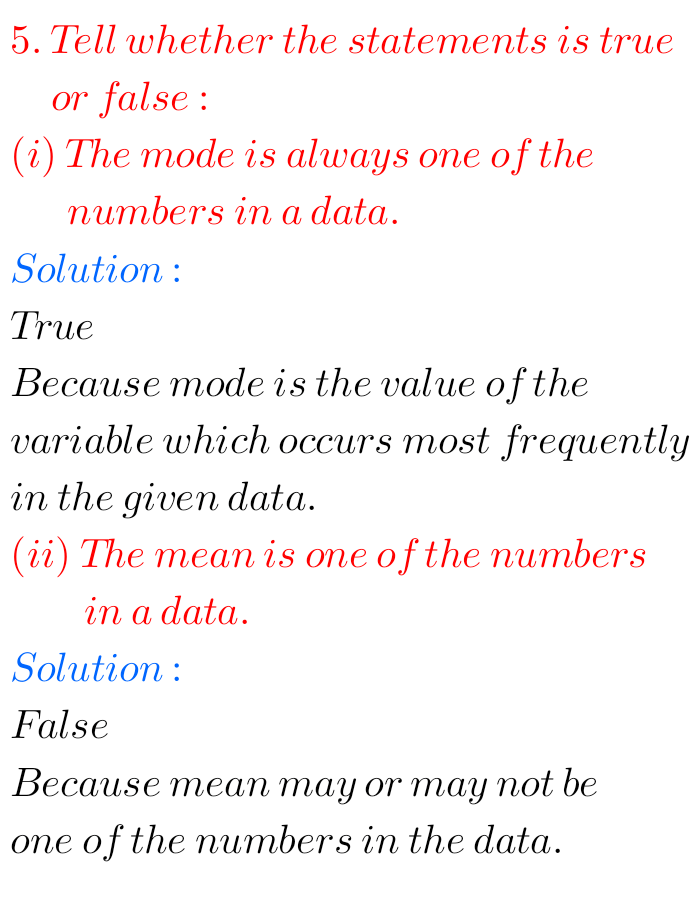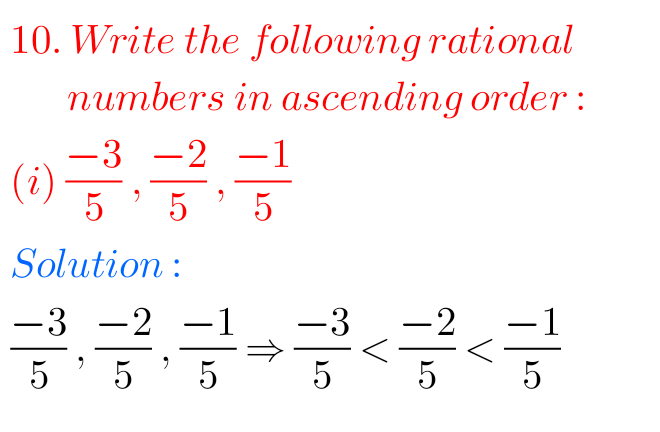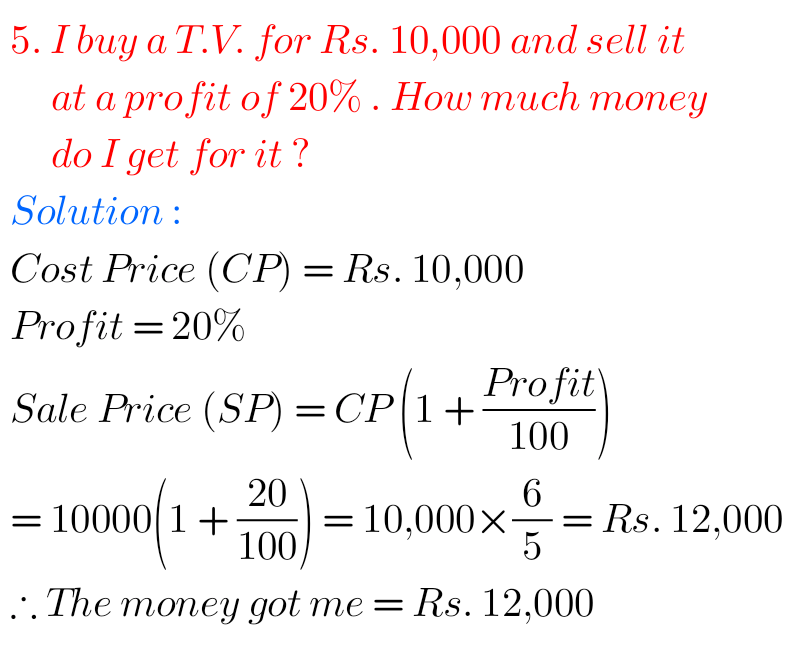# ncert solutions for class 7th mathematics## NCERT solutions for Maths Class 7 Chapter 3 Data Handling Exercise 3.2

Data Handling Exercise 3.2 solutions class 7 maths NCERT m NCERT mathematics class 7 Data Handling Chapter 3 exercise 3.2 solutions are given. You should study the lesson Data Handling very well. You should also practice all example problems and solutions given in the textbook. Observe the solutions and try them in your own method. …

## NCERT maths solutions for Class 7 Chapter 3 Data Handling Exercise 3.1

Data Handling exercise 3.1 solutions NCERT maths class 7 NCERT mathematics class 7 Data Handling Chapter 3 exercise 3.1 solutions are given. You should study the textbook lesson Data Handling very well. You should also practice all example problems and solutions given in the textbook. Observe the solutions and try them in your own method. …

## NCERT solutions for class 7 exercise 8.2 Rational Numbers Maths

Rational Numbers exercise 8.2 solutions class 7 chapter 8 NCERT mathematics class 7 Rational Numbers Chapter 8 exercise 8.2 solutions are given. You should study the lesson Rational Numbers very well. You should also practice all example problems and solutions given in the textbook. Observe the solutions and try them in your own method. m …## NCERT maths solutions for class 7 chapter 8 exercise 8.1 Rational Numbers

Rational numbers exercise 8.1 solutions class 7 NCERT NCERT mathematics class 7 Rational Numbers Chapter 8 exercise 8.1 solutions are given. You should study the lesson Rational Numbers very well. You should also practice all example problems and solutions given in the textbook. Observe the solutions and try them in your own method. m Maths …## NCERT solutions for maths class 7 chapter 7 exercise 7.2 Comparing Quantities

Comparing Quantities exercise 7.2 solutions NCERT maths class 7 NCERT mathematics class 7 chapter 7.2 exercise 7.2 solutions are given. You should read the textbook lesson Comparing Quantities very well. You should also practice all example problems and solutions given in the textbook. Observe the solutions and try them in your own method. NCERT solutions …## NCERT maths solutions for class 7 chapter 7 Comparing Quantities exercise 7.1

Comparing Quantities solutions exercise 7.1 class 7 maths NCERT NCERT Mathematics class 7 chapter 7 exercise 7.1 Comparing Quantities solutions are given. You should study the lesson Comparing Quantities very well. You should observe and practice all example problems and solutions given in the textbook. NCERT solutions for class 6 NCERT solutions for  class 7 …

## NCERT solutions for class 7th maths chapter 2/Fractions and Decimals solutions

NCERT SOLUTIONS FOR CLASS 7TH MATHS CHAPTER 2 FRACTIONS AND DECIMALS Fractions and Decimals Chapter 2 class 7 Maths NCERT solutions are given. You should study the textbook lesson Fractions and Decimals very well. You should observe and practice the example problems and solutions given in the textbook. Observe the given below solutions and try …## Ncert solutions for class 7th maths chapter 4 Simple Equations, problems with solutions for Simple Equations vii class cbse

NCERT SOLUTIONS FOR CLASS 7 TH MATHS CHAPTER 4, SIMPLE EQUATIONS Simple Equations class 7-chapter 4 maths NCERT solutions are given. You should study the textbook lesson Simple Equations very well. You should observe and practice the example problems and solutions given in the textbook. Observe the given below solutions and try them in your …

## Ncert solutions for class 7th maths chapter 1/Integers solutions,problems with solutions of Integers vii class

NCERT SOLUTIONS FOR CLASS 7th MATHS CHAPTER 1,INTEGERS SOLUTIONS Integers class 7 chapter 1 Ncert Maths solutions are given. Study the textbook lesson very well. Observe and practice example problems and solutions given in the textbook. CBSE SOLUTIONS FOR CLASS 7th MATHS CHAPTER 1,SOLUTIONS FOR INTEGERS Important points 1. Integers are closed under addition. Integers …